# DAG scheduling and timetables in Airflow

One of the fundamental features of Apache Airflow is the ability to schedule jobs. Historically, Airflow users scheduled their DAGs by specifying a `schedule` with a cron expression, a timedelta object, or a preset Airflow schedule. Timetables, released in Airflow 2.2, allow users to create their own custom schedules using Python, effectively eliminating the limitations of cron. With timetables, you can now schedule DAGs to run at any time. Datasets, introduced in Airflow 2.4, let you schedule your DAGs on updates to a dataset rather than a time-based schedule. For more information about datasets, see Datasets and Data-Aware Scheduling in Airflow.

In this guide, you'll learn Airflow scheduling concepts and the different ways you can schedule a DAG with a focus on timetables. For a video overview of these concepts, see Scheduling in Airflow webinar.

All code used in this guide is available in the airflow-scheduling-tutorial repository.

## Assumed knowledge​

To get the most out of this guide, you should have an existing knowledge of:

## Scheduling concepts​

To gain a better understanding of DAG scheduling, it's important that you become familiar with the following terms and parameters:

• Data Interval: A property of each DAG run that represents the period of data that each task should operate on. For example, for a DAG scheduled hourly, each data interval begins at the top of the hour (minute 0) and ends at the close of the hour (minute 59). The DAG run is typically executed at the end of the data interval.
• Logical Date: The start of the data interval. It does not represent when the DAG will be executed. Prior to Airflow 2.2, this was referred to as the execution date.
• Timetable: A DAG property that dictates the data interval and logical date for each DAG run and determines when a DAG is scheduled.
• Run After: The earliest time the DAG can be scheduled. This date is shown in the Airflow UI, and may be the same as the end of the data interval depending on your DAG's timetable.
• Backfilling and Catchup: Related to scheduling. To learn more, see DAG Runs.

The `execution_date` concept was deprecated in Airflow 2.2. If you're using an older versions of Airflow and need more information about `execution_date`, see What does execution_date mean?.

### Parameters​

The following parameters ensure your DAGs run at the correct time:

• `data_interval_start`: Defines the start date and time of the data interval. A DAG's timetable will return this parameter for each DAG run. This parameter is created automatically by Airflow, or is specified by the user when implementing a custom timetable.
• `data_interval_end`: Defines the end date and time of the data interval. A DAG's timetable will return this parameter for each DAG run. This parameter is created automatically by Airflow, or is specified by the user when implementing a custom timetable.
• `schedule`: Defines when a DAG will be run. This value is set at the DAG configuration level. It accepts cron expressions, timedelta objects, timetables, and lists of datasets.
• `start_date`: The first date your DAG will be executed. This parameter is required for your DAG to be scheduled by Airflow.
• `end_date`: The last date your DAG will be executed. This parameter is optional.

In Airflow 2.3 and earlier, the `schedule_interval` is used instead of the `schedule` parameter and it only accepts cron expressions or timedelta objects. Additionally, timetables have to be passed using the `timetable` parameter, which was deprecated in Airflow 2.4 and later. In versions of Airflow 2.2 and earlier, specifying `schedule_interval` is the only way to define a DAG schedule.

### Example​

To demonstrate how these concepts work together, consider a DAG that is scheduled to run every 5 minutes. Looking at the most recent DAG run, the logical date is `2022-08-28 22:37:33`, which is displayed in the Data interval start field in the following image. The logical date is also included in the Run ID field and identifies the DAG run in the Airflow metadata database. The value in the Data interval end field is 5 minutes later.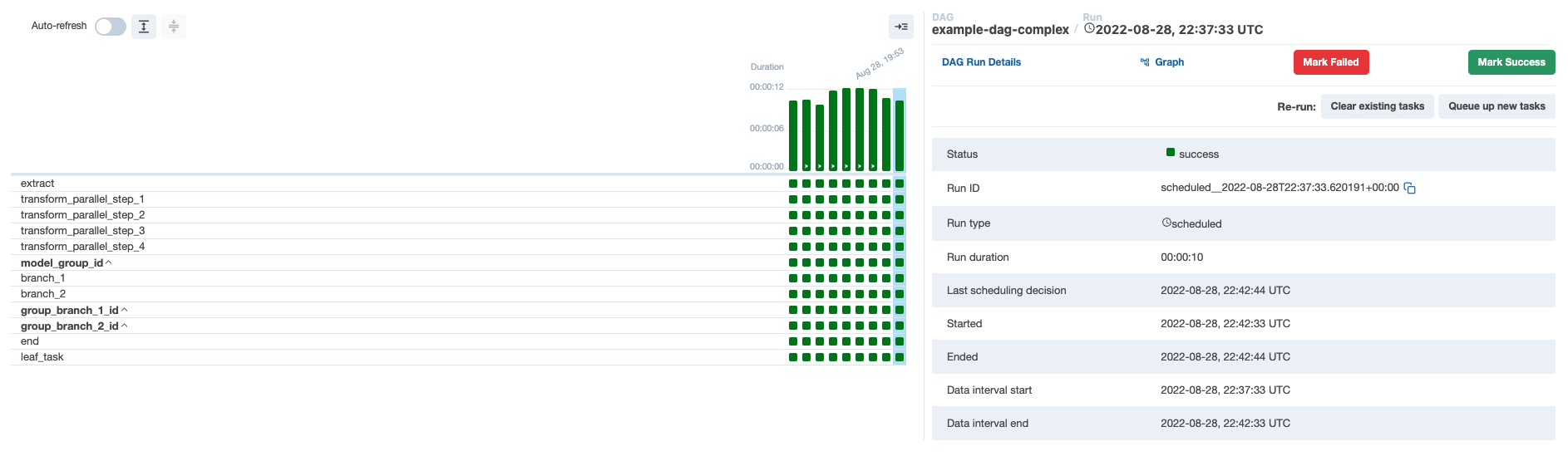If you look at the next DAG run in the UI, the logical date is `2022-08-28 22:42:33`, which is shown as the Next Run value in the Airflow UI. This is 5 minutes after the previous logical date, and the same value shown in the Data interval end field of the previous DAG run. If you hover over Next Run, you can see that the Run After value, which is the date and time that the next DAG run will actually start, matches the value in the Data interval end field: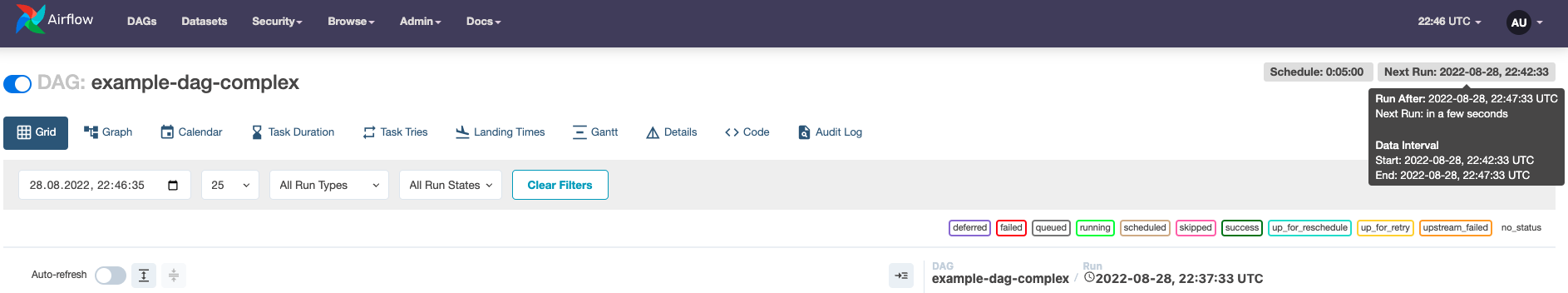The following is a comparison of the two successive DAG runs:

• DAG run 1 (`scheduled__2022-08-28T22:37:33.620191+00:00`) has a logical date of `2022-08-28 22:37:33`, a data interval start of `2022-08-28 22:37:33` and a data interval end of `2022-08-28 22:42:33`. This DAG run will actually start at `2022-08-28 22:42:33`.
• DAG run 2 (`scheduled__2022-08-28T22:42:33.617231+00:00`) has a logical date of `2022-08-28 22:42:33`, a data interval start of `2022-08-28 22:42:33` and a data interval end of `2022-08-28 22:47:33`. This DAG run will actually start at `2022-08-28 22:47:33`.

## Cron-based schedules​

For pipelines with straightforward scheduling needs, you can define a `schedule` in your DAG using:

• A cron expression.
• A cron preset.
• A timedelta object.

### Setting a cron-based schedule​

#### Cron expressions​

You can pass any cron expression as a string to the `schedule` parameter in your DAG. For example, if you want to schedule your DAG at 4:05 AM every day, you would use `schedule='5 4 * * *'`.

If you need help creating the correct cron expression, see crontab guru.

#### Cron presets​

Airflow can utilize cron presets for common, basic schedules. For example, `schedule='@hourly'` will schedule the DAG to run at the beginning of every hour. For the full list of presets, see Cron Presets. If your DAG does not need to run on a schedule and will only be triggered manually or externally triggered by another process, you can set `schedule=None`.

#### Timedelta objects​

If you want to schedule your DAG on a particular cadence (hourly, every 5 minutes, etc.) rather than at a specific time, you can pass a `timedelta` object imported from the `datetime` package to the `schedule` parameter. For example, `schedule=timedelta(minutes=30)` will run the DAG every thirty minutes, and `schedule=timedelta(days=1)` will run the DAG every day.

Note: Do not make your DAG's schedule dynamic (e.g. `datetime.now()`)! This will cause an error in the Scheduler.

### Cron-based schedules and the logical date​

Airflow was originally developed for extract, transform, and load (ETL) with the expectation that data is constantly flowing in from some source and then will be summarized at a regular interval. However, if you want to summarize data from Monday, you need to wait until Tuesday at 12:01 AM. This shortcoming led to the introduction of timetables in Airflow 2.2.

Each DAG run has a `logical_date` that is separate from the time that the DAG run is expected to begin. A DAG run is not actually allowed to run until the `logical_date` for the following DAG run has passed. So, if you're running a daily DAG, the Monday DAG run will not execute until Tuesday. In this example, the `logical_date` is Monday 12:01 AM, even though the DAG run will not actually begin until Tuesday 12:01 AM.

If you want to pass a timestamp to the DAG run that represents the earliest time at which this DAG run can started, use `{{ next_ds }}` from the jinja templating macros.

Astronomer recommends that you make each DAG run idempotent (able to be re-run without changing the result) which precludes using `datetime.now()`.

### Limitations of cron-based schedules​

The relationship between a DAG's `schedule` and its `logical_date` leads to particularly unintuitive results when the spacing between DAG runs is irregular. The most common example of irregular spacing is when DAGs run only during business days from Monday to Friday. In this case, the DAG run with a Friday `logical_date` will not run until Monday, even though the data from Friday is available on Saturday. A DAG that summarizes results at the end of each business day can't be set using only `schedule`. In Airflow 2.2 and earlier, you must schedule the DAG to run every day (including Saturday and Sunday) and include logic in the DAG to skip all tasks on the days the DAG doesn't need to run.

The following are the limitations of a traditional schedule:

• Schedule a DAG at different times on different days. For example, 2:00 PM on Thursdays and 4:00 PM on Saturdays.
• Schedule a DAG daily except for holidays.
• Schedule a DAG at multiple times daily with uneven intervals. For example, 1:00 PM and 4:30 PM.

These limitations were addressed in Airflow 2.2 and later with the introduction of timetables.

## Timetables​

Timetables, introduced in Airflow 2.2, address the limitations of cron expressions and timedelta objects by allowing users to define their own schedules in Python code. All DAG schedules are ultimately determined by their internal timetable and if a cron expression or timedelta object is not suitable, you can define your own.

Custom timetables can be registered as part of an Airflow plugin. They must be a subclass of `Timetable`, and they should contain the following methods, both of which return a `DataInterval` with a start and an end:

• `next_dagrun_info`: Returns the data interval for the DAG's regular schedule
• `infer_manual_data_interval`: Returns the data interval when the DAG is manually triggered

### Continuous timetable​

As of Airflow 2.6, you can run a DAG continuously with a pre-defined timetable. To use the ContinuousTimetable, set the schedule of your DAG to `"@continuous"` and set `max_active_runs` to 1.

``@dag(    start_date=datetime(2023, 4, 18),    schedule="@continuous",    max_active_runs=1,      catchup=False,)``

This schedule will create one continuous DAG run, with a new run starting as soon as the previous run has completed, regardless of whether the previous run succeeded or failed. Using a ContinuousTimetable is especially useful when sensors or deferrable operators are used to wait for highly irregular events in external data tools.

caution

Airflow is designed to handle orchestration of data pipelines in batches, and this feature is not intended for streaming or low-latency processes. If you need to run pipelines more frequently than every minute, consider using Airflow in combination with tools designed specifically for that purpose like Apache Kafka.

### Example custom timetable​

For this implementation, you'll run your DAG at 6:00 and 16:30. Because this schedule has run times with differing hours and minutes, it can't be represented by a single cron expression. So, you'll implement this schedule with a custom timetable.

To start, you need to define the `next_dagrun_info` and `infer_manual_data_interval` methods. The time the DAG runs (`run_after`) should be the end of the data interval since the interval doesn't have any gaps. To run a DAG that at 6:00 and 16:30, you have the following alternating intervals:

• Run at 6:00: Data interval is from 16:30 on the previous day* to 6:00 on the current day.
• Run at 16:30: Data interval is from 6:00 to 16:30 on the current day.

You define the `next_dagrun_info` method to provide Airflow with the logic to calculate the data interval for scheduled runs. The method also contains logic to handle the DAG's `start_date`, `end_date`, and `catchup` parameters. To implement the logic in this method, you use the Pendulum package. The method is shown in the following example:

``def next_dagrun_info(    self,    *,    last_automated_data_interval: Optional[DataInterval],    restriction: TimeRestriction,) -> Optional[DagRunInfo]:    if last_automated_data_interval is not None:  # There was a previous run on the regular schedule.        last_start = last_automated_data_interval.start        delta = timedelta(days=1)        if last_start.hour == 6: # If previous period started at 6:00, next period will start at 16:30 and end at 6:00 following day            next_start = last_start.set(hour=16, minute=30).replace(tzinfo=UTC)            next_end = (last_start+delta).replace(tzinfo=UTC)        else: # If previous period started at 16:30, next period will start at 6:00 next day and end at 16:30            next_start = (last_start+delta).set(hour=6, minute=0).replace(tzinfo=UTC)            next_end = (last_start+delta).replace(tzinfo=UTC)    else:  # This is the first ever run on the regular schedule. First data interval will always start at 6:00 and end at 16:30        next_start = restriction.earliest        if next_start is None:  # No start_date. Don't schedule.            return None        if not restriction.catchup: # If the DAG has catchup=False, today is the earliest to consider.            next_start = max(next_start, DateTime.combine(Date.today(), Time.min).replace(tzinfo=UTC))        next_start = next_start.set(hour=6, minute=0).replace(tzinfo=UTC)        next_end = next_start.set(hour=16, minute=30).replace(tzinfo=UTC)    if restriction.latest is not None and next_start > restriction.latest:        return None  # Over the DAG's scheduled end; don't schedule.    return DagRunInfo.interval(start=next_start, end=next_end)``

The code example completes the following process:

• If there was a previous run for the DAG:
• If the previous DAG run started at 6:00, then the next DAG run should start at 16:30 and end at 6:00 the next day.
• If the previous DAG run started at 16:30, then the DAG run should start at 6:00 the next day and end at 16:30 the next day.
• If it is the first run of the DAG:
• Check for a start date. If there isn't one, the DAG can't be scheduled.
• Check if `catchup=False`. If so, the earliest date to consider should be the current date. Otherwise it is the DAG's start date.
• The first DAG run should always start at 6:00. So, update the time of the interval start to 6:00 and the end to 16:30.
• If the DAG has an end date, do not schedule the DAG after that date has passed.

Now, you define the data interval for manually triggered DAG runs by defining the `infer_manual_data_interval` method. The code appears similar to the following example:

``def infer_manual_data_interval(self, run_after: DateTime) -> DataInterval:    delta = timedelta(days=1)    # If time is between 6:00 and 16:30, period ends at 6am and starts at 16:30 previous day    if run_after >= run_after.set(hour=6, minute=0) and run_after <= run_after.set(hour=16, minute=30):        start = (run_after-delta).set(hour=16, minute=30, second=0).replace(tzinfo=UTC)        end = run_after.set(hour=6, minute=0, second=0).replace(tzinfo=UTC)    # If time is after 16:30 but before midnight, period is between 6:00 and 16:30 the same day    elif run_after >= run_after.set(hour=16, minute=30) and run_after.hour <= 23:        start = run_after.set(hour=6, minute=0, second=0).replace(tzinfo=UTC)        end = run_after.set(hour=16, minute=30, second=0).replace(tzinfo=UTC)    # If time is after midnight but before 6:00, period is between 6:00 and 16:30 the previous day    else:        start = (run_after-delta).set(hour=6, minute=0).replace(tzinfo=UTC)        end = (run_after-delta).set(hour=16, minute=30).replace(tzinfo=UTC)    return DataInterval(start=start, end=end)``

This method determines what the most recent complete data interval is based on the current time. The following are the possible outcomes:

• The current time is between 6:00 and 16:30: In this case, the data interval is from 16:30 the previous day to 6:00 the current day.
• The current time is after 16:30 but before midnight: In this case, the data interval is from 6:00 to 16:30 the current day.
• The current time is after midnight but before 6:00: In this case, the data interval is from 6:00 to 16:30 the previous day.

Three sets of logic are required to account for time periods in the same timeframe (6:00 to 16:30) on different days than the day that the DAG is triggered. When you define custom timetables, keep in mind what the last complete data interval should be based on when the DAG should run.

Now, you combine the two methods in a `Timetable` class which will make up your Airflow plugin. The following example is a full custom timetable plugin:

``from datetime import timedeltafrom typing import Optionalfrom pendulum import Date, DateTime, Time, timezonefrom airflow.plugins_manager import AirflowPluginfrom airflow.timetables.base import DagRunInfo, DataInterval, TimeRestriction, TimetableUTC = timezone("UTC")class UnevenIntervalsTimetable(Timetable):    def infer_manual_data_interval(self, run_after: DateTime) -> DataInterval:        delta = timedelta(days=1)        # If time is between 6:00 and 16:30, period ends at 6am and starts at 16:30 previous day        if run_after >= run_after.set(hour=6, minute=0) and run_after <= run_after.set(hour=16, minute=30):            start = (run_after-delta).set(hour=16, minute=30, second=0).replace(tzinfo=UTC)            end = run_after.set(hour=6, minute=0, second=0).replace(tzinfo=UTC)        # If time is after 16:30 but before midnight, period is between 6:00 and 16:30 the same day        elif run_after >= run_after.set(hour=16, minute=30) and run_after.hour <= 23:            start = run_after.set(hour=6, minute=0, second=0).replace(tzinfo=UTC)            end = run_after.set(hour=16, minute=30, second=0).replace(tzinfo=UTC)        # If time is after midnight but before 6:00, period is between 6:00 and 16:30 the previous day        else:            start = (run_after-delta).set(hour=6, minute=0).replace(tzinfo=UTC)            end = (run_after-delta).set(hour=16, minute=30).replace(tzinfo=UTC)        return DataInterval(start=start, end=end)    def next_dagrun_info(        self,        *,        last_automated_data_interval: Optional[DataInterval],        restriction: TimeRestriction,    ) -> Optional[DagRunInfo]:        if last_automated_data_interval is not None:  # There was a previous run on the regular schedule.            last_start = last_automated_data_interval.start            delta = timedelta(days=1)            if last_start.hour == 6: # If previous period started at 6:00, next period will start at 16:30 and end at 6:00 following day                next_start = last_start.set(hour=16, minute=30).replace(tzinfo=UTC)                next_end = (last_start+delta).replace(tzinfo=UTC)            else: # If previous period started at 14:30, next period will start at 6:00 next day and end at 14:30                next_start = (last_start+delta).set(hour=6, minute=0).replace(tzinfo=UTC)                next_end = (last_start+delta).replace(tzinfo=UTC)        else:  # This is the first ever run on the regular schedule. First data interval will always start at 6:00 and end at 16:30            next_start = restriction.earliest            if next_start is None:  # No start_date. Don't schedule.                return None            if not restriction.catchup: # If the DAG has catchup=False, today is the earliest to consider.                next_start = max(next_start, DateTime.combine(Date.today(), Time.min).replace(tzinfo=UTC))            next_start = next_start.set(hour=6, minute=0).replace(tzinfo=UTC)            next_end = next_start.set(hour=16, minute=30).replace(tzinfo=UTC)        if restriction.latest is not None and next_start > restriction.latest:            return None  # Over the DAG's scheduled end; don't schedule.        return DagRunInfo.interval(start=next_start, end=next_end)class UnevenIntervalsTimetablePlugin(AirflowPlugin):    name = "uneven_intervals_timetable_plugin"    timetables = [UnevenIntervalsTimetable]``

Because timetables are plugins, you'll need to restart the Airflow Scheduler and Webserver after adding or updating them.

In the DAG, you can import the custom timetable plugin and use it to schedule the DAG by setting the `schedule` parameter (in pre-2.4 Airflow you will need to use the `timetable` parameter):

``from uneven_intervals_timetable import UnevenIntervalsTimetable@dag(    dag_id="example_timetable_dag",    start_date=datetime(2021, 10, 9),    max_active_runs=1,    schedule=UnevenIntervalsTimetable(),    default_args={        "retries": 1,        "retry_delay": duration(minutes=3),    },    catchup=True)``

Looking at the Tree View in the UI, you can see that this DAG has run twice per day at 6:00 and 16:30 since the start date of 2021-10-09.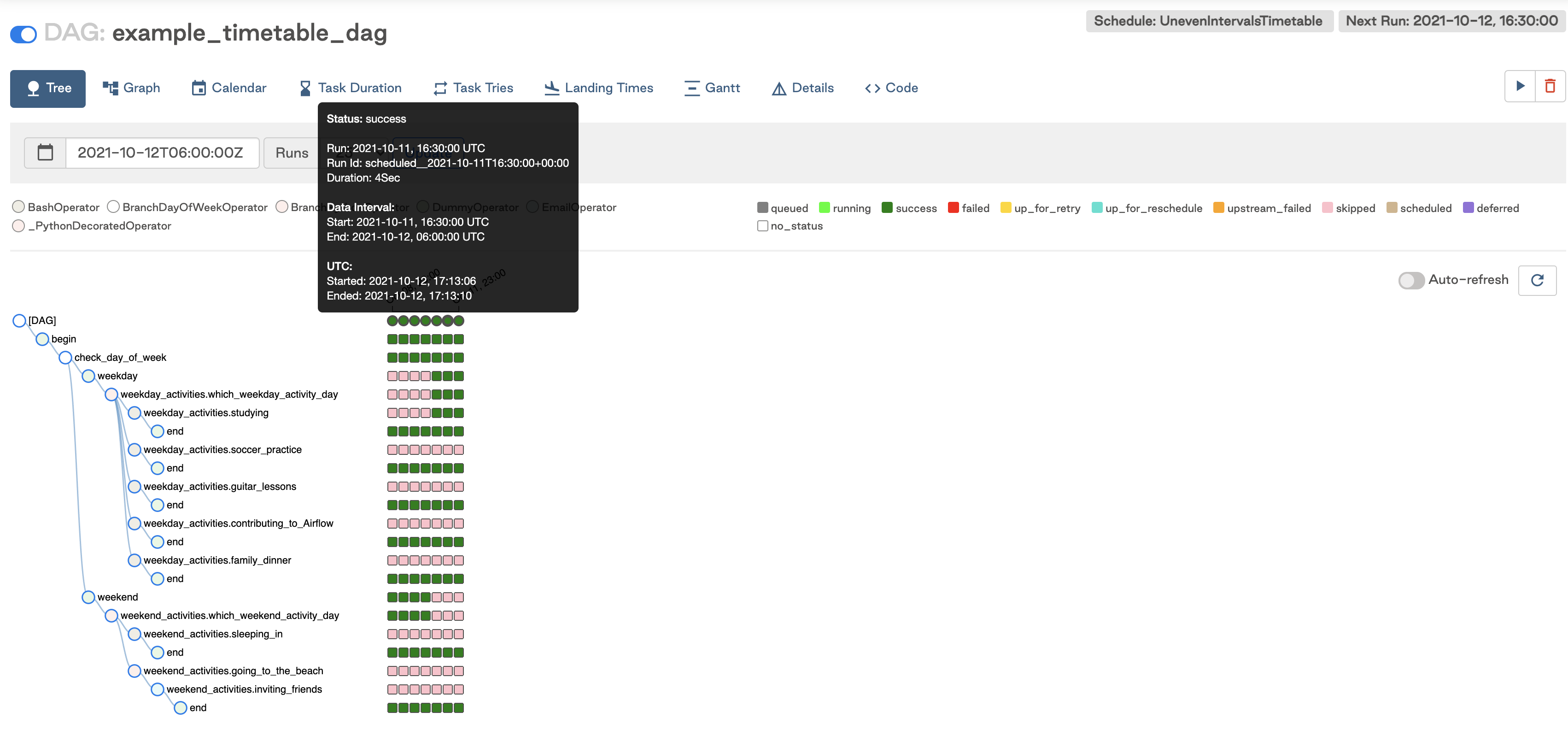The next scheduled run is for the interval starting on 2021-10-12 at 16:30 and ending the following day at 6:00. This run will be triggered at the end of the data interval, so after 2021-10-13 6:00.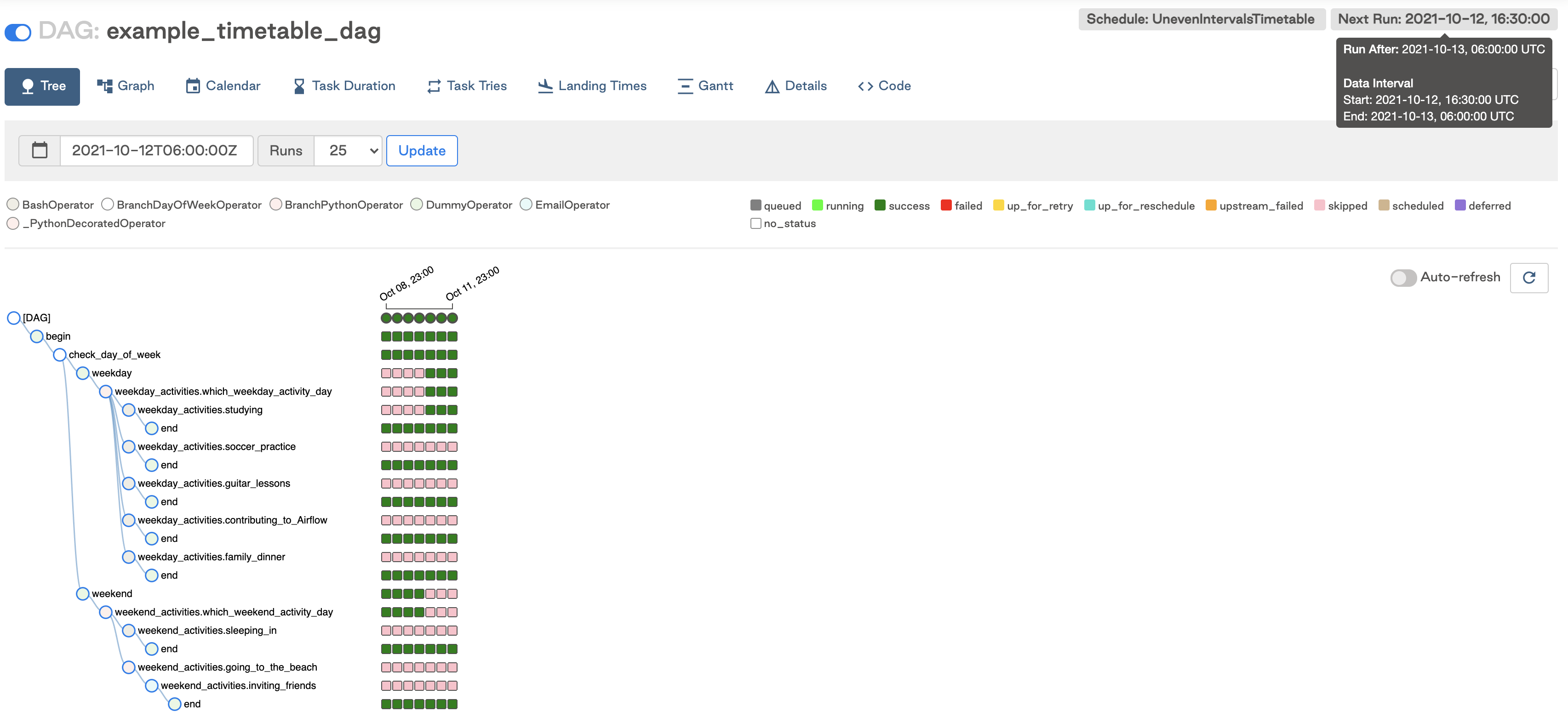If you run the DAG manually after 16:30 but before midnight, you can see the data interval for the triggered run was between 6:00 and 16:30 that day as expected.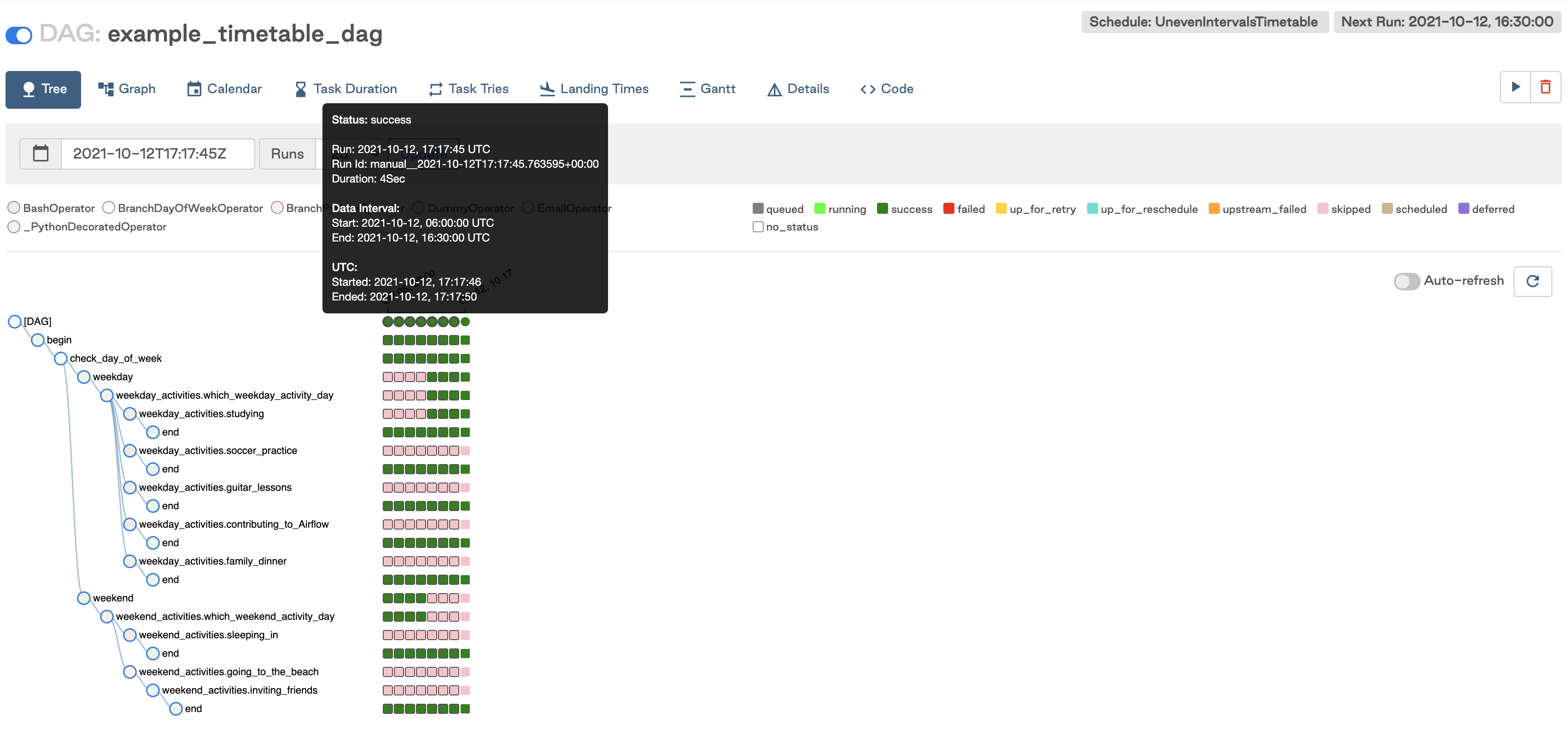This timetable can be adjusted to suit other use cases. Timetables are customizable as long as the methods above are implemented.

When you implement your timetable logic, make sure that your `next_dagrun_info` method does not return a `data_interval_start` that is earlier than your DAG's `start_date`. This will result in tasks not being executed for that DAG run.

### Current limitations​

There are some limitations to keep in mind when implementing custom timetables:

• Timetable methods should return the same result every time they are called (e.g. avoid things like HTTP requests). They are not designed to implement event-based triggering.
• Timetables are parsed by the scheduler when creating DAG runs, so avoid slow or lengthy code that could impact Airflow performance.

## Dataset driven scheduling​

Datasets and data-driven DAG dependencies were introduced in Airflow 2.4. You can now make Airflow detect when a task in a DAG updates a data object. Using that awareness, other DAGs can be scheduled depending on updates to these datasets. To create a dataset-based schedule, you pass the names of the datasets as a list to the `schedule` parameter. For example:

``dataset1 = Dataset(f"{DATASETS_PATH}/dataset_1.txt")dataset2 = Dataset(f"{DATASETS_PATH}/dataset_2.txt")@dag(    dag_id='dataset_dependent_example_dag',    catchup=False,    start_date=datetime(2022, 8, 1),    schedule=[dataset1, dataset2],    tags=['consumes', 'dataset-scheduled'],)``

This DAG runs only when `dataset1` and `dataset2` are updated. These updates can occur by tasks in different DAGs as long as they are located in the same Airflow environment.

In the Airflow UI, the DAG now has a schedule of Dataset and the Next Run column shows how many datasets the DAG depends on and how many of them have been updated.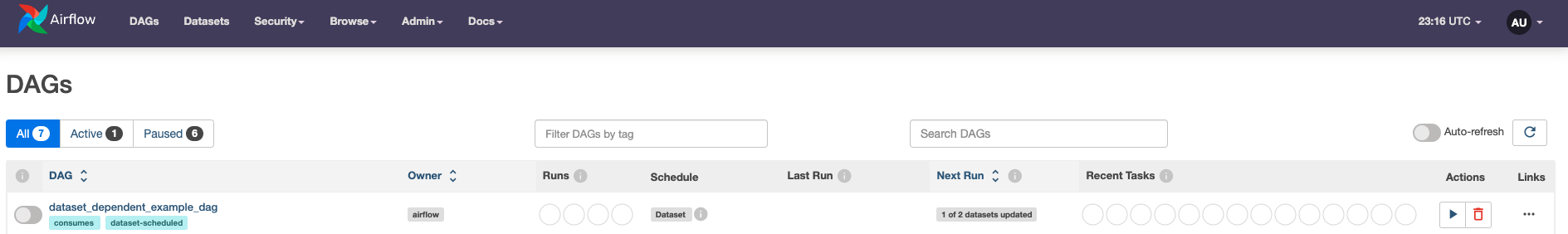To learn more about datasets and data driven scheduling, see Datasets and Data-Aware Scheduling in Airflow guide.